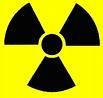Science Quiz / 'I' & 'J' Units of Measurement

Random Science or Computer Quiz

Can you name the units of measurement that begin with 'i' or 'j?

byCryptus  Plays Quiz not verified by Sporcle

Score
0/8
Timer
06:00
ClueUnit
A wine bottle measure, equal to 6 litres.
A unit of length, equal to 1/12 of a foot.
A unit used in radio astronomy to measure the strength of radio signals from space.
A unit used in high energy physics, equivalent to a gigajoule; it is also the name for a change in acceleration.
A wine bottle measure, equal to 3 litres.
A unit of time, defined as the length of one cycle, or tick, of a computer's system clock.
A unit of volume for liquor, generally considered equal to 1.5 fluid ounces.
The SI unit of work or energy, defined as the work done by a force of 1 newton acting to move an object through a distance of 1 metre in the direction in which the force is applied

From the Vault See Another

Movies at the Half-Hour Mark (2010s)

by aglick

Movies 5m
By the half hour mark you've probably decided whether or not you care about the movie.

Extras

Created Jan 13, 2016